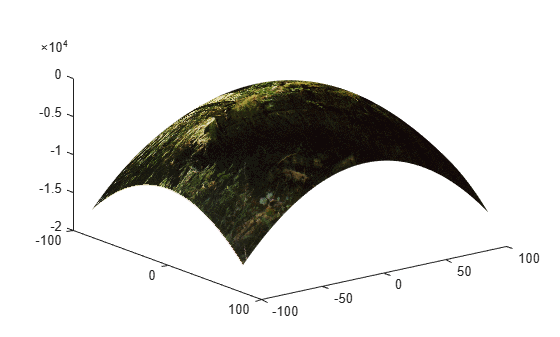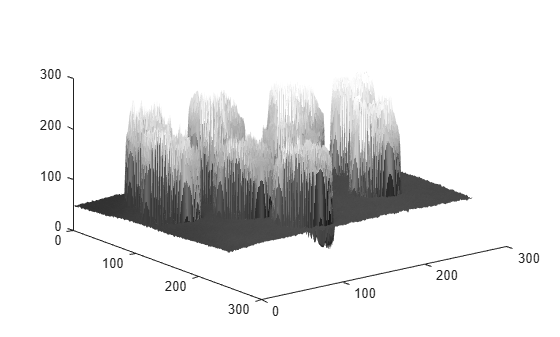# warp

Display image as texture-mapped surface

## Syntax

``warp(X,map)``
``warp(I,n)``
``warp(BW)``
``warp(RGB)``
``warp(Z,___)``
``warp(X,Y,Z,___)``
``h = warp(___)``

## Description

````warp(X,map)` displays the indexed image `X` with colormap `map` as a texture map on a simple rectangular surface.```
````warp(I,n)` displays the intensity image `I` with `n` levels as a texture map on a simple rectangular surface.```
````warp(BW)` displays the binary image `BW` as a texture map on a simple rectangular surface.```
````warp(RGB)` displays the truecolor image `RGB` as a texture map on a simple rectangular surface.```

example

````warp(Z,___)` displays the image on the surface `Z`.```

example

````warp(X,Y,Z,___)` displays the image on the surface `(X,Y,Z)`.```
````h = warp(___)` returns the texture-mapped surface.```

## Examples

collapse all

This example shows how to warp an indexed image over a nonuniform surface. This example uses a curved surface centered at the origin.

Read an indexed image into the workspace.

`[I,map] = imread('forest.tif');`

Create the surface. First, define the x- and y-coordinates of the surface. This example uses arbitrary coordinates that are unrelated to the indexed image. Note that the size of the coordinate matrices `X` and `Y` do not need to match the size of the image.

`[X,Y] = meshgrid(-100:100,-80:80);`

Define the height `Z` of the surface at the coordinates given by (`X,Y`).

`Z = -(X.^2 + Y.^2);`

Warp the image over the surface defined by the coordinates (`X,Y,Z`).

```figure warp(X,Y,Z,I,map);```Explore the warped image interactively using the rotate and data cursor tools.

Read a grayscale image into the workspace.

`I = imread('coins.png');`

Warp the image over the surface whose height is equal to the intensity of the image `I`. Specify the number of graylevels.

```figure warp(I,I,128);```Note that the x- and y-coordinates of the surface were not specified in the call to `warp` and thus default to the image pixel indices. Explore the warped image interactively using the rotate and data cursor tools.

## Input Arguments

collapse all

Indexed image, specified as a 2-D numeric matrix. The values in `X` are an index into `map`, an n-by-3 array of RGB values.

Data Types: `single` | `double` | `uint8` | `uint16` | `int16` | `logical`

Colormap, specified as an n-by-3 numeric matrix. Each row specifies an RGB color value. When `map` is type `single` or `double`, values must be in the range [0, 1].

Data Types: `single` | `double` | `uint8`

Grayscale image, specified as a 2-D numeric matrix.

Data Types: `single` | `double` | `uint8` | `uint16` | `int16` | `logical`

Number of grayscale levels, specified as a positive integer.

Data Types: `double` | `uint8` | `uint16` | `logical`

Binary image, specified as a 2-D logical matrix.

Data Types: `logical`

Truecolor image, specified as an m-by-n-by-3 numeric array.

Data Types: `single` | `double` | `uint8` | `uint16` | `int16` | `logical`

Height of surface, specified as a 2-D numeric matrix. When `Z` is not specified, the surface is flat with a uniform height of 0.

Data Types: `single` | `double` | `uint8` | `uint16` | `uint32` | `uint64` | `int8` | `int16` | `int32` | `int64` | `logical`

x-coordinates of surface, specified as a 2-D numeric matrix.

Data Types: `single` | `double` | `uint8` | `uint16` | `uint32` | `uint64` | `int8` | `int16` | `int32` | `int64` | `logical`

y-coordinates of surface, specified as a 2-D numeric matrix.

Data Types: `single` | `double` | `uint8` | `uint16` | `uint32` | `uint64` | `int8` | `int16` | `int32` | `int64` | `logical`

## Output Arguments

collapse all

Texture-mapped surface, returned as a `Surface` object. For more information, see Surface Properties.

## Tips

• Texture-mapped surfaces are generally rendered more slowly than images.

• The `warp` function sets the `YDir` axes property to `"reverse"`. Values along the y-axis increase from top to bottom. To decrease the values from top to bottom, set `YDir` to `"normal"`. This setting reverses both the y-axis and the image.

## Version History

Introduced before R2006a